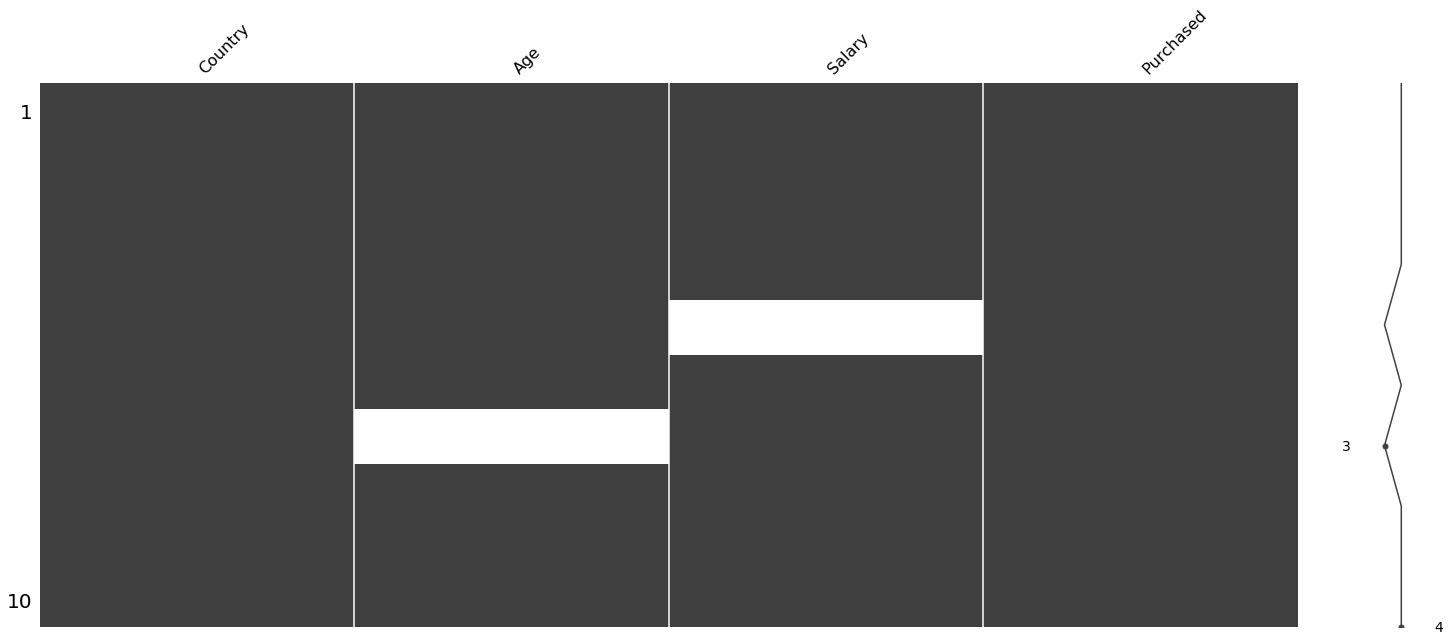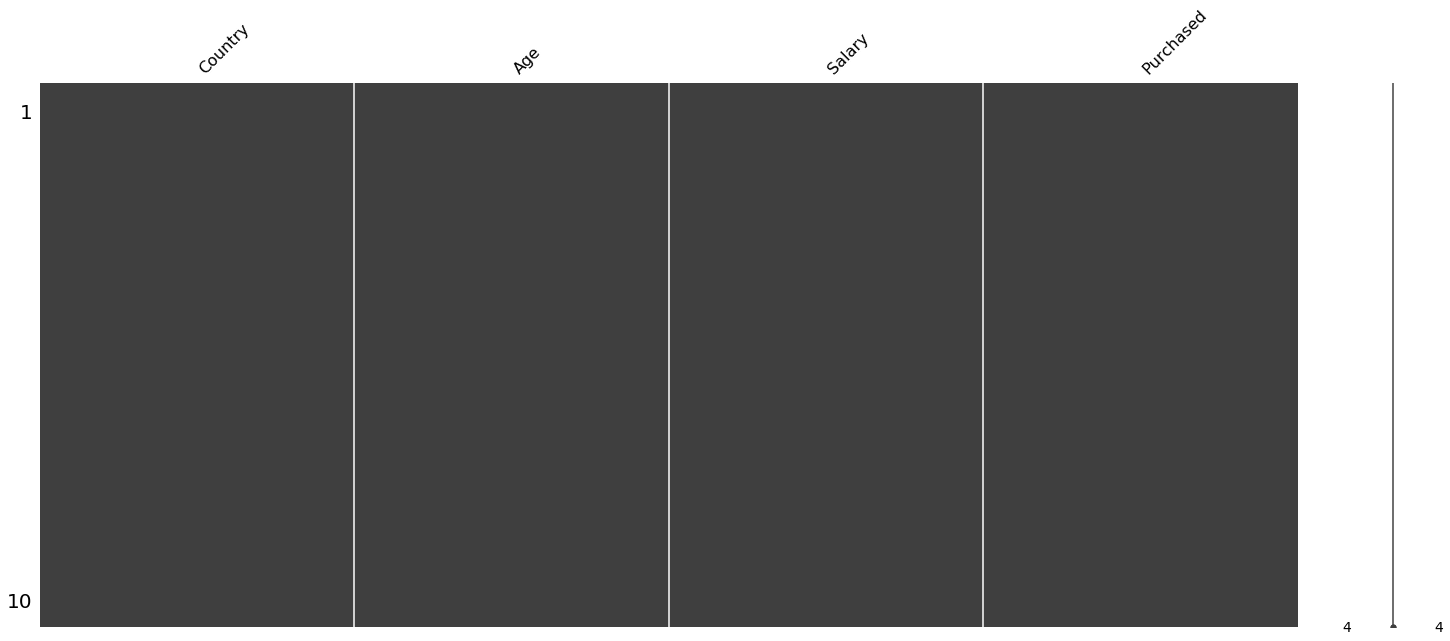0%

This Blog Will Be Focusing On The Preprocessing The Data Mostly EDA, Finding Missing Values, Filling and Feature Scaling

• Importing Libraries
• Creating Dataset
• EDA on Dataset
• Find Missing Values
• Replace The Missing Values
• Visualizing Data
• Categorical variables
• Splitting the dataset to train and test dataset
• Feature Scaling

## Importing Libraries

In :
import pandas as pd
import numpy as np
from matplotlib import pyplot as plt
import random


## Creating Dataset

In :
df = pd.DataFrame({"Country": ['France', 'Spain', 'Germany', 'Spain', 'Germany', 'France', 'Spain', 'Germany', 'Spain', 'France'], 'Age': [44, 27, 30, 38, 40 , 35, np.nan, 48 , 50 , 37], 'Salary': [72000, 48000, 54000, 61000, np.nan, 58000, 52000, 79000, 83000, 67000], 'Purchased': ['No','Yes', 'No', 'No', 'Yes', 'Yes', 'No', 'Yes', 'No', 'Yes' ]})


## EDA on Dataset

In :
print(df.info())

<class 'pandas.core.frame.DataFrame'>
RangeIndex: 10 entries, 0 to 9
Data columns (total 4 columns):
#   Column     Non-Null Count  Dtype
---  ------     --------------  -----
0   Country    10 non-null     object
1   Age        9 non-null      float64
2   Salary     9 non-null      float64
3   Purchased  10 non-null     object
dtypes: float64(2), object(2)
memory usage: 448.0+ bytes
None

In :
print(df.head(4))

   Country   Age   Salary Purchased
0   France  44.0  72000.0        No
1    Spain  27.0  48000.0       Yes
2  Germany  30.0  54000.0        No
3    Spain  38.0  61000.0        No

In :
print(df.describe())

             Age        Salary
count   9.000000      9.000000
mean   38.777778  63777.777778
std     7.693793  12265.579662
min    27.000000  48000.000000
25%    35.000000  54000.000000
50%    38.000000  61000.000000
75%    44.000000  72000.000000
max    50.000000  83000.000000


## Find Missing Values

### Missing Values: We Have Some Missing Values. We will find them first.

In :
import missingno as mp
mp.matrix(df) #will plot the missing value chart

Out:
<matplotlib.axes._subplots.AxesSubplot at 0x1a204dd910>In :
print(df.isna().sum()) #will print the total of null values

Country      0
Age          1
Salary       1
Purchased    0
dtype: int64


## Replace The Missing Values

In :
df= df.fillna(df.mean()) #replace the missing values with the mean of the values


### Let us visualize again and check if the missing values are filled or not

In :
mp.matrix(df) #will plot the missing value chart

Out:
<matplotlib.axes._subplots.AxesSubplot at 0x1a241a1150>##### We can also use imputer to do the same task

Here is the Code for the same:

from sklearn.impute import SimpleImputer
imputer = SimpleImputer(missing_values = np.nan, strategy = 'mean',verbose=0)

imputer = imputer.fit(X.iloc[:, 1:3])
X.iloc[:, 1:3] = imputer.transform(X.iloc[:, 1:3])

imputer = imputer.fit(df.iloc[: , 1:3])

df.iloc[:,1:3] = imputer.transform(df.iloc[:, 1:3])

.

### Deviding the dataset first to dependent and indepandent values

In :
x = df.iloc[: , 0:3].values # independent variable
y = df.iloc[: , 3:4].values # dependent variable

In :
print(x)

[['France' 44.0 72000.0]
['Spain' 27.0 48000.0]
['Germany' 30.0 54000.0]
['Spain' 38.0 61000.0]
['Germany' 40.0 63777.77777777778]
['France' 35.0 58000.0]
['Spain' 38.77777777777778 52000.0]
['Germany' 48.0 79000.0]
['Spain' 50.0 83000.0]
['France' 37.0 67000.0]]

In :
print(y)

[['No']
['Yes']
['No']
['No']
['Yes']
['Yes']
['No']
['Yes']
['No']
['Yes']]


..

## Categorical variables

#### One hot encoder is used to make a sparse matrix of the numerical features encoded by Cloumn Transformer. It encodes into 0 and 1 Sparse Matrix

.

In :
from sklearn.compose import ColumnTransformer
from sklearn.preprocessing import OneHotEncoder
ct = ColumnTransformer(transformers = [('encoder', OneHotEncoder(), )], remainder = 'passthrough') #creating an instance
x = np.array(ct.fit_transform(x))

In :
print(x)

[[1.0 0.0 0.0 44.0 72000.0]
[0.0 0.0 1.0 27.0 48000.0]
[0.0 1.0 0.0 30.0 54000.0]
[0.0 0.0 1.0 38.0 61000.0]
[0.0 1.0 0.0 40.0 63777.77777777778]
[1.0 0.0 0.0 35.0 58000.0]
[0.0 0.0 1.0 38.77777777777778 52000.0]
[0.0 1.0 0.0 48.0 79000.0]
[0.0 0.0 1.0 50.0 83000.0]
[1.0 0.0 0.0 37.0 67000.0]]

In :
print(y)

[['No']
['Yes']
['No']
['No']
['Yes']
['Yes']
['No']
['Yes']
['No']
['Yes']]


.

#### It is preferred to use label encoder to change the binary data so we use LabelEncoder

In :
#it is preferred to use label encoder to change the binary data so we use LabelEncoder
from sklearn.preprocessing import LabelEncoder
encoder = LabelEncoder()
y = encoder.fit_transform(y)
y = np.reshape(y , (10,1))

/Users/karan7k/opt/anaconda3/lib/python3.7/site-packages/sklearn/preprocessing/_label.py:251: DataConversionWarning: A column-vector y was passed when a 1d array was expected. Please change the shape of y to (n_samples, ), for example using ravel().
y = column_or_1d(y, warn=True)


## Splitting the dataset into training set and test set

In :
from sklearn.model_selection import train_test_split
x_train , x_test , y_train , y_test = train_test_split(x, y , test_size = 0.3 , random_state = 0)

In :
print('x_train: ', x_train, '\n', 'y_train: ', y_train, '\n' ,'x_test: ', x_test, '\n', 'y_test: ', y_test)

x_train:  [[1.0 0.0 0.0 37.0 67000.0]
[0.0 0.0 1.0 27.0 48000.0]
[0.0 0.0 1.0 38.77777777777778 52000.0]
[0.0 1.0 0.0 48.0 79000.0]
[0.0 0.0 1.0 38.0 61000.0]
[1.0 0.0 0.0 44.0 72000.0]
[1.0 0.0 0.0 35.0 58000.0]]
y_train:  [





]
x_test:  [[0.0 1.0 0.0 30.0 54000.0]
[0.0 0.0 1.0 50.0 83000.0]
[0.0 1.0 0.0 40.0 63777.77777777778]]
y_test:  [

]


### We can also use numpy method too

#### ! DO NOT RUN THIS PART IF YOU ARE USING ABOVE METHOD

In :
random.seed(2) # so that we get the same results
splt = np.random.rand(len(df)) < 0.8 #80, 20 split or random
x_train , y_train = x[splt] , y[splt]
x_test , y_test = x[~splt], y[~splt]

In :
print('x_train: ', x_train, '\n', 'y_train: ', y_train, '\n' ,'x_test: ', x_test, '\n', 'y_test: ', y_test)

x_train:  [[0.0 0.0 1.0 27.0 48000.0]
[0.0 1.0 0.0 30.0 54000.0]
[0.0 0.0 1.0 38.0 61000.0]
[0.0 1.0 0.0 40.0 63777.77777777778]
[1.0 0.0 0.0 35.0 58000.0]
[0.0 0.0 1.0 38.77777777777778 52000.0]
[1.0 0.0 0.0 37.0 67000.0]]
y_train:  [





]
x_test:  [[1.0 0.0 0.0 44.0 72000.0]
[0.0 1.0 0.0 48.0 79000.0]
[0.0 0.0 1.0 50.0 83000.0]]
y_test:  [

]


## Feature Scaling

#### We have 2 important parts in feature scaling

• Standardization
• Normalization

The terms normalization and standardization are sometimes used interchangeably, but they usually refer to different things.

Standardization rescales data to have a mean (𝜇) of 0 and standard deviation (𝜎) of 1 (unit variance). Formulae for Standardization is $X_{changed} = \frac{X - \mu}{\sigma}$ For most applications standardization is recommended.

Normalization usually means to scale a variable to have a values between 0 and 1, while standardization transforms data to have a mean of zero and a standard deviation of 1.

Normalization rescales the values into a range of [0,1]. This might be useful in some cases where all parameters need to have the same positive scale. However, the outliers from the data set are lost. Formulae for normalization is $X_{changed} = \frac{X - X_{min}}{X_{max}-X_{min}}$

In :
from sklearn.preprocessing import StandardScaler
sc = StandardScaler()


We must standardize the data only after the split and the scaler should be only be fitted to the x_train set because if we do that we get the mean and standard of the values in the x_test which should be hidden to us. So we will only fit the scaler to the test set and then we will transform the scaler to x_test </p>

#### Do we have to apply/standardization to the dummy variables(Country Column here) to the matrix of features ?

Simply as the goal of Standardization is to transform your data and get them in the range generally (-3 , +3) But here we have mostly the data in 0s and 1s after we have converted them using ColumnTransformer, OneHotEncoder and LabelEncoder.

And there is nothing extra to be done here.
Moreever here standardization will convert the values to -3 and +3 which will worsen out understanding of the data as we will not be able to understand the nonsense numerical values.

Feature Scaling on the dataset makes the model better but when we do the same on the dummy variabe it makes the data not redable and we can not relate the country to the salary etc.
So feature Scaling should be applyed to the model but not to the dummy variable as they are already encoded before using ColumnTransformer, OneHotEncoder and LabelEncoder.

### Transforming the non dummy values in train set after fitting

#### Therefore we should only apply feature scaling to the non dummy values ie the values that are numbers

In :
x_train[: , 3:] = sc.fit_transform(x_train[:, 3:])

In :
print(x_train)

[[0.0 0.0 1.0 -1.8060709482255894 -1.5457062438816012]
[0.0 1.0 0.0 -1.13807210436133 -0.5878751616074286]
[0.0 0.0 1.0 0.6432581459433618 0.5295944343791061]
[0.0 1.0 0.0 1.0885907085195348 0.9730347502467791]
[1.0 0.0 0.0 -0.02474069792089762 0.0506788932420198]
[0.0 0.0 1.0 0.8164430313896515 -0.9071521890321528]
[1.0 0.0 0.0 0.42059186465527537 1.4874255166532786]]


### Transforming the non dummy values in test set

##### We will not fit the test dataset as we want that dataset to be unknown, Therefore we will only transform this dataset
In :
x_test[: , 3:] = sc.transform(x_test[:, 3:])

In :
print(x_test)

[[1.0 0.0 0.0 1.9792558336718808 2.285618085215089]
[0.0 1.0 0.0 2.8699209588242267 3.4030876812016237]
[0.0 0.0 1.0 3.3152535214003995 4.041641736051072]]